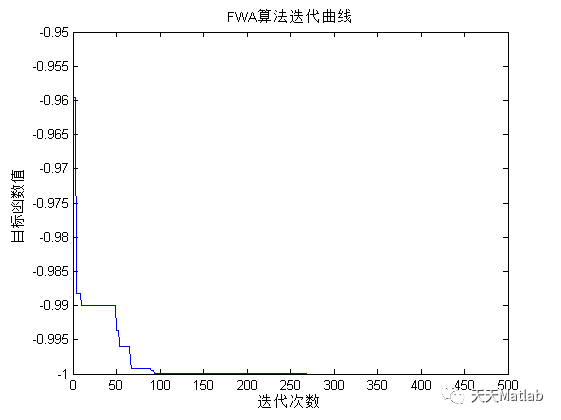# 【智能优化算法-烟花算法】基于烟花算法求解单目标优化问题含Matlab源码

+关注继续查看

✅作者简介：热爱科研的Matlab仿真开发者，修心和技术同步精进，matlab项目合作可私信。

🍎个人主页：Matlab科研工作室

🍊个人信条：格物致知。

## 2 部分代码

%% 烟花算法进行函数优化

%%求函数的最小值

%fitness适应度函数，N烟花数，D变量维数，M变异火花数，Er爆炸半径，En爆炸数目

%LB,UB分别为变量上下界，T为迭代次数，a,b为爆炸数目限制因子

clear;clc

close all

N=100;   % N烟花数

D=2;     % D变量维数

M=5;     % M变异火花数

En=6;    % En爆炸数目

Er=5;    % Er爆炸半径

a=0.3;   % a,b为爆炸数目限制因子

b=0.6;

T=500;   % T为迭代次数

%求最大值变量上下界

LB=[-10,-10];

UB=[10,10];

%求最大值输出

[F(T),Y]=min(Fit);

fmin=min(F);

xm=x(Y,:);

fprintf('xm=%f  %f\n',xm(1),xm(2));

fprintf('fmin=%f\n',fmin);

figure(1);

t=1:T;

plot(t,F)

xlabel('迭代次数')

ylabel('目标函数值')

title('FWA算法迭代曲线');

## 3 运行结果编辑

## 4 参考文献

杨彦龙向淑文夏顺友贾文生. 基于烟花算法的非合作博弈Nash均衡问题求解[J]. 计算机应用与软件, 2018, 035(003):215-218.

❤️ 关注我领取海量matlab电子书和数学建模资料

❤️部分理论引用网络文献，若有侵权联系博主删除

|
13小时前
|

【MATLAB 】 EMD信号分解+FFT傅里叶频谱变换组合算法
【MATLAB 】 EMD信号分解+FFT傅里叶频谱变换组合算法
9 0
|
13小时前
|

【MATLAB 】 EEMD信号分解+FFT傅里叶频谱变换组合算法
【MATLAB 】 EEMD信号分解+FFT傅里叶频谱变换组合算法
14 0
|
13小时前
|

【MATLAB】 CEEMD信号分解+FFT傅里叶频谱变换组合算法
【MATLAB】 CEEMD信号分解+FFT傅里叶频谱变换组合算法
8 0
|
13小时前
|

【MATLAB】 CEEMDAN信号分解+FFT傅里叶频谱变换组合算法
【MATLAB】 CEEMDAN信号分解+FFT傅里叶频谱变换组合算法
9 0
|
13小时前
|

【MATLAB】 ICEEMDAN信号分解+FFT傅里叶频谱变换组合算法
【MATLAB】 ICEEMDAN信号分解+FFT傅里叶频谱变换组合算法
8 0
|
13小时前
|

【MATLAB】 小波分解信号分解+FFT傅里叶频谱变换组合算法
【MATLAB】 小波分解信号分解+FFT傅里叶频谱变换组合算法
10 0
|
13小时前
|

【MATLAB】 LMD信号分解+FFT傅里叶频谱变换组合算法
【MATLAB】 LMD信号分解+FFT傅里叶频谱变换组合算法
12 0
|
13小时前
|

【MATLAB】 RLMD信号分解+FFT傅里叶频谱变换组合算法
【MATLAB】 RLMD信号分解+FFT傅里叶频谱变换组合算法
8 0
|
13小时前
|

【MATLAB】mlptdenoise信号分解+FFT傅里叶频谱变换组合算法
【MATLAB】mlptdenoise信号分解+FFT傅里叶频谱变换组合算法
12 0
|
13小时前
|

【MATLAB】 MODWT信号分解+FFT傅里叶频谱变换组合算法
【MATLAB】 MODWT信号分解+FFT傅里叶频谱变换组合算法
10 0